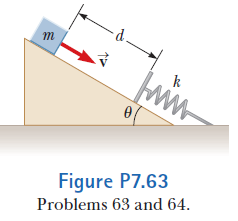Problem: An inclined plane of angle θ = 20.0° has a spring of force constant k = 500 N/m fastened securely at the bottom so that the spring is parallel to the surface as shown in Figure P7.63. A block of mass m = 2.50 kg is placed on the plane at a distance d = 0.300 m from the spring. From this position, the block is projected downward toward the spring with speed v = 0.750 m/s. By what distance is the spring compressed when the block momentarily comes to rest?

FREE Expert Solution
84% (497 ratings)
FREE Expert Solution

We'll use the law of conservation of energy.

$\overline{){{\mathbf{E}}}_{{\mathbf{i}}}{\mathbf{=}}{{\mathbf{E}}}_{{\mathbf{f}}}}$

84% (497 ratings)
Problem Details

An inclined plane of angle θ = 20.0° has a spring of force constant k = 500 N/m fastened securely at the bottom so that the spring is parallel to the surface as shown in Figure P7.63. A block of mass m = 2.50 kg is placed on the plane at a distance d = 0.300 m from the spring. From this position, the block is projected downward toward the spring with speed v = 0.750 m/s. By what distance is the spring compressed when the block momentarily comes to rest?